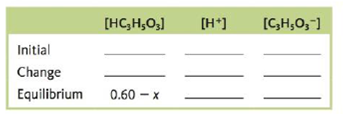Chapter 14, Problem 164CWP

Chapter
Section
Textbook Problem

# Consider a 0.60-M solution of HC3H5O3, lactic acid (Ka = 1.4 × 10−4).a. Which of the following are major species in the solution?i. HC3H5O3ii. C3H5O3−iii. H+iv. H2Ov. OH−b. Complete the following ICE table in terms of x, the amount (mol/L) of lactic acid that dissociates to reach equilibrium.c. What is the equilibrium concentration for C3H3O3−?d. Calculate the pH of the solution.

(a)

Interpretation Introduction

Interpretation: The major species in the given solution of Lactic acid (CH3CH(OH)COOH) , missing values in the stated ICE table, the equilibrium concentration of C3H5O3 and the pH of solution is to be stated.

Concept introduction: Lactic acid is a α- hydroxyl acid and is more acidic than acetic acid due to the intramolecular hydrogen bonding. It is a chiral compound and dissociates in water as a weak acid.

To determine: The major species in the given solution of Lactic acid (CH3CH(OH)COOH) .

Explanation

Explanation

The major species in solution are lactate ion (C3H5O3) and H+ .

The dissociation reaction of Lactic acid in aqueous solution is,

CH3

(b)

Interpretation Introduction

Interpretation: The major species in the given solution of Lactic acid (CH3CH(OH)COOH) , missing values in the stated ICE table, the equilibrium concentration of C3H5O3 and the pH of solution is to be stated.

Concept introduction: Lactic acid is a α- hydroxyl acid and is more acidic than acetic acid due to the intramolecular hydrogen bonding. It is a chiral compound and dissociates in water as a weak acid.

To determine: The missing values in the given ICE table.

(c)

Interpretation Introduction

Interpretation: The major species in the given solution of Lactic acid (CH3CH(OH)COOH) , missing values in the stated ICE table, the equilibrium concentration of C3H5O3 and the pH of solution is to be stated.

Concept introduction: Lactic acid is a α- hydroxyl acid and is more acidic than acetic acid due to the intramolecular hydrogen bonding. It is a chiral compound and dissociates in water as a weak acid.

To determine: The equilibrium concentration of C3H5O3 .

(d)

Interpretation Introduction

Interpretation: The major species in the given solution of Lactic acid (CH3CH(OH)COOH) , missing values in the stated ICE table, the equilibrium concentration of C3H5O3 and the pH of solution is to be stated.

Concept introduction: Lactic acid is a α- hydroxyl acid and is more acidic than acetic acid due to the intramolecular hydrogen bonding. It is a chiral compound and dissociates in water as a weak acid.

### Still sussing out bartleby?

Check out a sample textbook solution.

See a sample solution

#### The Solution to Your Study Problems

Bartleby provides explanations to thousands of textbook problems written by our experts, many with advanced degrees!

Get Started